# Anticipating and Managing Critical Noise Sources In MEMS Gyroscopes

When a MEMS inertial measurement unit (IMU) is operating as a feedback sensor in a motion control system, gyroscope noise is an important behavior to understand because it can cause undesirable physical motion on the platform it is monitoring. Depending on the conditions, there are a number of potential error sources to consider when developing early, application-targeted noise estimates for a particular MEMS IMU. Three common gyroscope attributes to consider in this process are its inherent noise, response to linear vibration and misalignment errors. Figure 1 provides a simple model, which displays several attributes that are influential in evaluating each error source: source, sensor response, and filtering. This model provides the baseline for spectral analysis of each of these attributes.

### Inherent Sensor Noise

The inherent sensor noise represents the random variation in the gyroscope’s output when it is operating in static inertial and environmental conditions. MEMS IMU data sheets typically offer the rate noise density (RND) parameter to describe their gyroscope’s inherent noise, with respect to frequency. This parameter typically uses units of °/sec/√Hz and is a key part of predicting the inherent noise for a particular filter configuration. Equation 1 provides a simple way to estimate the noise associated with a particular frequency response (noise bandwidth) and RND.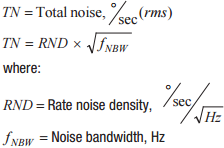When the RND’s frequency response follows a single-pole or double-pole, low-pass filter profile, the noise bandwidth (fNBW) relates to the filter cutoff frequency (fC) according to Equation 2 and Equation 3.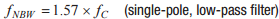In addition to the RND parameter, MEMS IMU data sheets sometimes specify the gyroscope’s inherent noise for a specific filter configuration using parameters such as output noise. This parameter typically uses standard units for angular rate (°/sec) and uses statistical terms, such as root-mean-square (rms), to describe the total noise magnitude.

### Linear Vibration

Since gyroscopes measure angular rate of rotation, their response to linear motion introduces error to their measurements. MEMS IMU data sheets typically describe this response to linear motion through parameters such as linear acceleration effect on bias or linear-g, which typically uses units of °/sec/g. Linear vibration is a repetitive inertial motion whose magnitude can be in terms of displacement (m), linear velocity (m/s), or linear acceleration (m/s2 or g). At a specific frequency of vibration (fLV), the displacement (|dLV|), velocity (|vLV|), and acceleration (|aLV|) magnitude relate to each other according to Equation 4.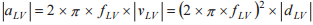When the vibration magnitude is in terms of acceleration (grms), multiply it by the linear-g parameter to estimate the resulting noise in the gyroscope measurements. For example, when the ADIS16488A experiences 5 g (rms) of vibration, the noise estimate in its gyroscopes will be 0.045°/sec (rms), since the linear-g is equal to 0.009 °/sec/g.As shown in Figure 1, gyroscope signal chains often include filters, which can help reduce the noise contribution from linear vibration. Defining the vibration in spectral terms (magnitude, frequency) provides an opportunity to consider the effect of the filters while estimating the noise contribution. The acceleration spectral density (ASD) function is a common way for expressing vibration in spectral terms and typically comes in units of g2/Hz. The following steps provide an example procedure for estimating the noise magnitude when the ASD and the gyroscope’s frequency response (HG) are known:

1. Multiply the ASD function by the square of the gyroscope’s frequency response: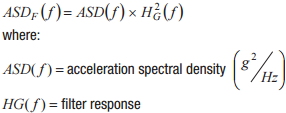2. Leverage Parseval’s theorem to calculate the average power in the “filtered vibration profile” by integrating ASDF over the frequency range of interest:3. Calculate the gyroscope noise magnitude by taking the square root of the noise power estimate and multiplying that result by the linear-g factor (HLG).### Misalignment Errors

Motion control systems typically establish an inertial reference frame, which contains three axes that are all 90° away from each other. These three axes provide an orientation reference for each sensor in a MEMS IMU. Ideally, each gyroscope’s axis of rotation will be perfectly aligned with its axis in the system’s reference frame, after installing the IMU onto the platform it will monitor during operation. In this scenario, rotation around one of the axes in the inertial reference frame will generate a response with only that axis’ gyroscope. In practice, this is not possible as mechanical imperfection inevitably causes some misalignment error, which causes the off-axis gyroscopes to respond to rotational motion around one axis in the inertial reference frame. Quantifying this response requires some trigonometric identities and careful definition of the gyroscope’s misalignment errors.

Each gyroscope’s misalignment error will have two components, each of which defines their alignment error with respect to another axis in the inertial reference frame. For example, in the system in Figure 2, θXZ represents the misalignment error of the x-axis gyroscope, with respect to z-axis. This misalignment error definition helps establish formulas for calculating the response of the off-axis gyroscope, to rotation around another axis in the system’s inertial reference frame. Equation 9 provides an example, which quantifies the response on the x-axis gyroscope (ωGX) due to its misalignment with respect to the z-axis (θXZ) and rotation around the z-axis (ωZR).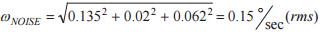MEMS IMUs typically have two types of alignment errors, which are related to each other, but have different applications for system-level modeling: axis-to-package and axis-to-axis. The axis-to-package misalignment error term describes the alignment of the gyroscopes with respect to specific mechanical features on the device package. When systems are not able to support inertial alignment after installing the IMU into the system, the axis-to-package misalignment error will be one of the key contributors to the overall misalignment errors. Mechanical imperfection in the system’s mechanical interface to the IMU can also contribute to the overall alignment error. The axis-to-axis misalignment error term describes the relative alignment accuracy of each gyroscope’s axis of rotation, with respect to the other two gyroscopes. This parameter is most influential when systems can implement a simple alignment process, which typically involves sensor observation while moving the entire assembly (IMU in place on the system platform) in a line, along one of the axes in the system’s inertial reference frame.

In cases where misalignment errors are not part of the IMU’s specifications, evaluating the physical tolerances of key mechanical features in device packages can provide some opportunity for estimating these errors. For example, the following scenarios introduce 0.5° of misalignment error:

• 35 μm of settling error in the solder reflow process on a 4 mm × 4 mm LGA package
• 0.175 mm of tolerance between two mounting holes that are 20 mm apart on a PCB

### Case Study

In order to illustrate these principles, consider the following example, which involves estimating the gyroscope noise in the ADIS16488A for new avionics system that operates under the following configuration/ conditions:

• Full bandwidth available from gyroscopes
• Vibration (ASD(f)): 0.122 g2/Hz; 10 Hz to 2000 Hz (Total vibration = 5 grms)
• Maximum rotational rate = ±100°/sec, frequency range = 5 Hz to 50 Hz

The full bandwidth configuration matches the conditions associated with the ADIS16488A’s output noise specification of 0.135°/sec (rms) for the inherent sensor noise. For the vibration contribution, Figure 3 provides an illustration of the profile, ASD(f), along with the filtered profile ASDF(f). The frequency response in ASDF(f) reflects the attenuation profile that is associated with the two-pole (404 Hz, 757 Hz), low-pass filter in this IMU’s gyroscope signal path.

Equation 10 multiplies the magnitude of the ASDF(f) profile, 2.24 grms, by the linear-g parameter to estimate the resulting noise level of 0.02°/sec (rms). This noise level is 55% lower than the 0.045°/sec (rms) level that came from Equation 5, which did not evaluate this noise source in spectral terms. This improvement is one example of deriving value from defining the vibration profile in spectral terms.Equation 11 provides a calculation for the x-axis gyroscope noise, which comes from a ±100°/sec rotational oscillation around the z-axis, at a frequency of 50 Hz. Since 50 Hz is well within the pass-band of the two-pole filter, the filter will provide no rejection of this noise source. This calculation assumes that the ADIS16488A’s axis-to-axis misalignment error will be the primary source of misalignment error (in other words, full deployment will include a simple inertial frame alignment process, after installing the IMU into the system).Table 1 summarizes the gyroscope noise from each attribute in the ADIS16488A. Equation 12 provides an overall noise estimate (ωNOISE) of 0.15°/sec (rms), which represents the root-sum-square (RSS) combination of all three noise sources in Table 1.

Table 1. Noise Contribution Summary

 Contribution Noise (°/sec) Inherent Sensor Noise 0.135 Linear vibration response 0.02 Misalignment 0.088### Conclusion

These techniques provide simple processes for evaluating common noise sources in MEMS gyroscope signals, using common parametric information in their data sheets, along with preliminary inertial condition insights or estimates. Understanding and evaluating these noise sources can help identify important application information, guide the IMU selection processes, and can reveal enhancement opportunities (simple alignment, when IMU supports appropriate axis-to-axis misalignment error levels) that may enable use of a more economical solution that would otherwise be available.

### Acknowledgements

The author would like to thank Randy Carver, Glenn Romano, Paul Perrault, and Dave LoCascio for their content contributions and review.